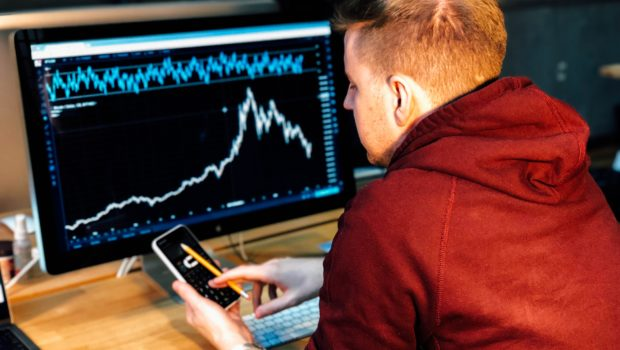FinancePublished on September 10th, 2020 | by Bibhuranjan

0

# Demystifying Forex Calculations – What is it and how is it done

Have you heard of the barter system, where you would take something in exchange for something else with the same value? Well, Forex is a kind of barter system that involves the exchange of currencies. This is like a trading platform for different currencies that can also be used for trading and commerce purposes.

This includes extensive technical calculations that are survey-based and factor-based.

Forex trading is a risky business as the currency pairs tend to fluctuate constantly. This makes it all the more important for traders to have a clear understanding of their Profits and Losses because it has a direct effect on their margin balance. If prices go against you, your margin balance will reduce, meaning that you will be left with less money for trading.

## Components of Forex Calculations

Forex calculations refer to the methods of calculating basic components of Forex trading. These methods have helped to reduce the difficulties that have arisen in forex trading over time. You do not obviously have to be a scientist or a mathematician to understand these formulae, but you are surely required to have the basic mathematical knowledge. The following are the basic components of forex calculations:-

1.Forex Margin

While trading, a trader must not invest all of his money; just a small amount will be fine. This is called a margin. How much margin is to be invested can be calculated by the formula:

Leverage is financial support from the broker.

Exchange rate of the currencies you are considering.

The margin in a forex account is called a performance bond as it is not borrowed money, but the equity needed to cover your losses.

2.Profit Calculator

This calculates the profit that the trader receives after investing; if the answer is negative, it means there is a loss. The formula for this is

Profit or Loss=(Price when selling the base currency – Price when buying the base currency)* Transaction size.

Transaction size is the price for carrying out the transaction.

3.CFD margin

This is the margin money to be invested for CFD, as they very risky to deal with, and the chances of loss are very high. The formula is

CFD margin =((Contract size*Market price)/(User Leverage))*Required margin

Rate.

Contract size: is the number of shares the trader is willing to trade.

Market price: is the market price of each share.

Prices of forex pairs are referred to in pairs, i.e., the bid price and the ask price. However, these two prices are not the same as the ask price. It is the price quoted on the right, which is usually higher than the bid price, which is quoted on the left. The difference in these two prices is known as the spread. This refers to the broker’s commission. The spread is measured in pips.

5. Pip

The value of a currency is given in pips in the forex market. One pip equals 0.0001, and two pips equals 0.0002, and so on.

6. Quotes

Quote is the price of a currency. Direct quotes and indirect quotes are two forms of quotes in the forex market.

A direct quote refers to the price for one US dollar in terms of another currency, whereas an indirect quote is a price for one UNIT of another currency in terms of the US dollar.

These are some of the known components of the Forex calculator; these are much more complex and advanced; however, one must be able to calculate the risks and profits of trading.

PRO’S

1. There are no external commissions except for the transaction cost, and is pretty feasible.
2. The Leverages provided here are pretty profitable here than the stock market as the ratio is 50:1 for Forex, whereas for other stock markets, it 2:1.

CON’S

1. When a huge loss is faced, you would have no idea about how to calculate leverage and other costs applied; the more the leverage, the higher the risk of loss.
2. No centralized system is used, and hence the broker manages all the funds, and all the calculations are done by him. This makes him the market maker.

## Conclusion

Forex is a good market for trading all kinds of currencies and trading activities. If you are experienced and intelligent enough to predict the calculations; you can earn a good profit. Still, you should also be able to predict the downfall or increment of a bid and also avoid scams.

Photo by Chris Liverani on Unsplash